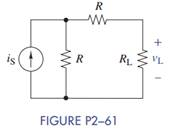Create an Account

Home / Questions / Use current division in Figure P2−61 to find an expression for v L in terms of R, R L , and i...

Use current division in Figure P2−61 to find an expression for v L in terms of R, R L , and i...

Use current division in Figure P2−61 to find an expression for vL in terms of R, RL, and iS.Jul 28 2020 View more View LessSubscribe To Get Solution